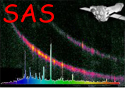XMM-Newton Science Analysis System

omdetect (omdetect-5.41) [xmmsas_20190531_1155-18.0.0]

## Point-like Sources (non-mosaiced images)

After the source-detection algorithms have finished, omdetect will compute raw count-rates for each detected source. For point-sources (ie those sources having widths consistent with those of the point-spread function) the count-rates are obtained by aperture photometry. For binned and unbinned images the aperture is 6 and 12 pixels, respectively. For a source with one or more neighbours that may be partly within the aperture, a smaller aperture will be used and its size is determined from the distance to its nearest neighbour. The actual aperture radii (unbinned pixels) that were used in the photometry are given in the column APERTURE of the SRCLIST table of the output source-list table.

The background is subtracted using the pixels within an annulus region. For the optical filters the inner and outer diameters of the annulus are 8 and 13 pixels for binned images, respectively, and 14 and 25 pixels for unbinned images, respectively. For the UV filters the inner and outer diameters of the annulus are 19.5 and 22 pixels for binned images, respectively, and 37 and 42 pixels for unbinned images, respectively. These values are stored as the fits keywords INNBCKAN and OUTBCKAN in the primary header of the output source-list file

When each point-source has had its photometry parameters computed, those with a significance of less than or equal to 10 sigma have their photometry re-computed using an aperture of 6 unbinned pixels, in order to reduce the errors due to the background noise.

These “raw” count-rates have to be corrected for coincidence-loss, dead-fraction and the time-dependent sensitivity degradation before instrumental magnitudes can be computed (using the task ommag). Please note that the time-dependent sensitivity and dead-time corrections are applied by ommag. The reference aperture through which XMM-OM coincidence-loss factors have been calibrated have a 12 pixel diameter. All raw count-rates obtained through reduced-size apertures are corrected to the reference aperture sizes using a calibrated PSF curves-of-growth, before coincidence-loss correction. The photometric zero-point calibration was performed on a 12 pixel diameter aperture in U, B and V filters, and a 35 pixel diameter one in UVW2, UVM2 and UVW1 filters. Therefore, UV point-source count-rates are extrapolated by curve-of-growth to 35 pixels before photometric calibration.

The following summarizes the procedure used to correct the source extracted count-rates for coincidence-losses-

• Extrapolate (if necessary) the source count-rate to the 12 unbinned pixel aperture, using the point-spread function for the filter.
• Extrapolate (if necessary) the background count-rate to the 12 unbinned pixel aperture, by using the scaling factor (144 / aperture).
• Apply a coincidence-loss correction to the total (source+background) count-rate.
• Apply a coincidence-loss correction to the background count-rate.
• Subtract the background coincidence-loss corrected count-rate from the coincidence-loss corrected total count-rate- this is the coincidence-corrected source-count rate.

Please note that these coincidence-loss corrections do not contain the factor 1/(1-deadfraction).

The following further corrections will then be applied by ommag-

• If a UV filter, a further point-spread function correction is applied to extrapolate the source count-rate from 12 to 35 unbinned pixels.